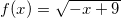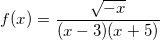# Domain and Range of a Function

A step by step tutorial, with detailed solutions, on how to find the domain and range of real valued functions is presented. First the definitions of these two concepts are presented. A table of domain and range of basic functions might be useful to answer the questions below.

## Definition of the Domain of a Function

For a function f defined by an expression with variable x, the implied domain of f is the set of all real numbers variable x can take such that the expression defining the function is real. The domain can also be given explicitly.
also
Step by Step Calculator to Find Domain of a Function

## Definition of the Range of a Function

The range of f is the set of all values that the function takes when x takes values in the domain.
Also a
Step by Step Calculator to Find Range of a Function is included in this website.

## Examples with Detailed Solutions

### Example 1

Find the domain of function f defined bySolution to Example 1
x can take any real number except 1 since x = 1 would make the denominator equal to zero and the division by zero is not allowed in mathematics. Hence the domain in interval notation is given by the set
(- ∞ , 1) U (1 , + ∞)

### Matched Problem 1

Find the domain of function f defined byAnswers to matched problems 1,2,3 and 4

### Example 2

Find the domain of function f defined bySolution to Example 2

The expression defining function f contains a square root. The expression under the radical has to satisfy the condition
2x - 8 >= 0
for the function to take real values.
Solve the above linear inequality
x >= 4
The domain, in interval notation, is given by
[4 , +∞)

### Matched Problem 2

Find the domain of function f defined by:### Example 3

Find the domain of function f defined by:Solution to Example 3
The expression defining function f contains a square root. The expression under the radical has to satisfy the condition
-x ≥ 0
Which is equivalent to
x ≤ 0
The denominator must not be zero, hence x not equal to 3 and x not equal to -5.
The domain of f is given  by
(-∞ , - 5) ∪ ( - 5 , 0]

### Matched Problem 3

Find the domain of function f defined by:### Example 4

Find the range of function f defined by:Solution to Example 4

The domain of this function is the set of all real numbers. The range is the set of values that f(x) takes as x varies. If x is a real number, x2 is either positive or zero. Hence we can write the following:
x
2 ≥ 0
Subtract - 2 to both sides to obtain
x
2  - 2 ≥ - 2
The last inequality indicates that x2 - 2 takes all values greater that or equal to - 2. The range of f is given by
[ -2 , +∞)
A graph of f also helps in interpreting the range of a function. Below is shown the graph of function f given above. Note the lowest point in the graph has a y (= f (x) ) value of - 2.### Matched Problem 4

Find the range of function f defined by: